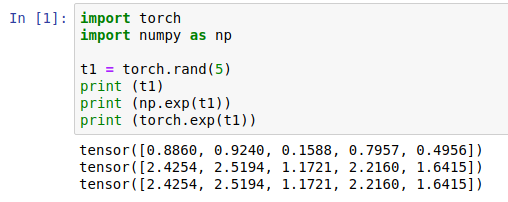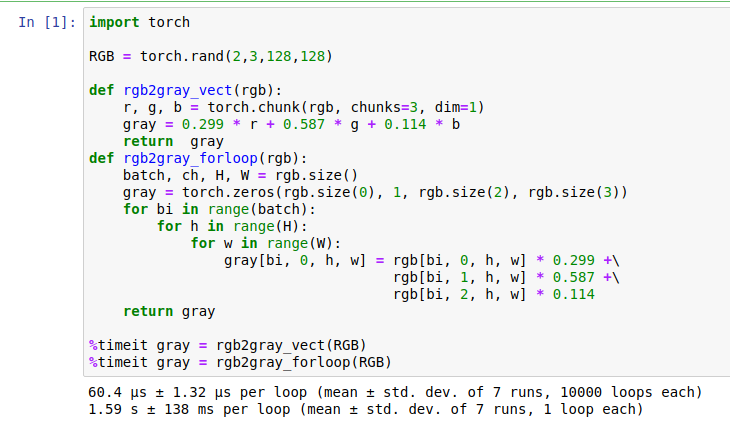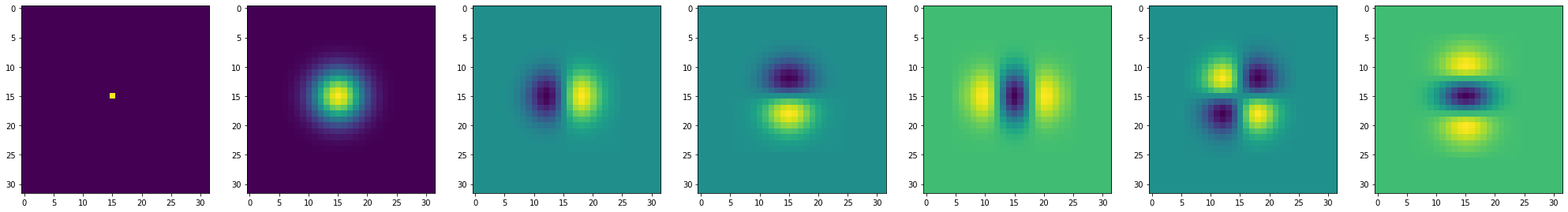Warning

# Introduction to Image Processing with Pytorch

Python, numpy and PyTorch will be used for the MPV labs. In case you are not familiar with them, study the following parts of the "Intro to numpy", "A Beginner-Friendly Guide to PyTorch and How it Works from Scratch", Pytorch for numpy users, |numpy for matlab users

To fulfil this assignment, you need to submit these files (all packed in one .zip file) into the upload system:

• imagefiltering.ipynb - a notebook for data initialisation, calling of the implemented functions and plotting of their results (for your convenience, will not be checked).
• imagefiltering.py - file with the following methods implemented:
• gaussian1d, gaussian_deriv1d - functions for computing Gaussian function and its first derivative.
• filter2d, - function for applying 2d filter kernel to image tensor
• gaussian_filter2d, spatial_gradient_first_order - functions for Gaussian blur and 1st order image spatial gradient computation
• affine, - function for transforming 3 points in the image into affine transformation matrix
• extract_affine_patches, - function extraction of the patches, defined by affine transform A.

Use template of the assignment. Use backup-template of the assignment.

When preparing a zip file for the upload system, do not include any directories, the files have to be in the zip file root.

# Basics of Image Processing in PyTorch

• To get the full usage of the parallel processing in PyTorch, the default choice is to work with 4d tensors of images. 4d tensor is an array of the shape [BxChxHxW], where B is batch size aka number of images, Ch is number of channels (3 for RGB, 1 for grayscale, etc.) H and W are height and width of the tensor.
• To convert image in form of numpy array (e.g., result of reading the image with OpenCV cv2.imread function), one could use function kornia.utils.image_to_tensor.
• PyTorch has a powerful autograd engine, which can be used for backpropagiting the error to the parameters and arguments. However, in the first part of this course we will not be using it, so one could save computation time and memory by running the functions under torch.no_grad()

import torch.nn.functional as F
out = F.conv2d(in, weight)

• PyTorch has two interfaces. One is object oriented and based on Modules, another is functional. Functional is more suitable for this course, although feel free to use modules, if it is more convenient to you.
• Remember, that you can use numpy functions on the pytorch tensors (only in CPU mode). Thus, if you are more familiar with numpy, you can use it for the labs.• Whenever possible, use vectorized operations instead of for-loops. For loops are very inefficient in python, pytorch nad matlab, unlike in C++, especially for images. See example below:## Convolution, Image Smoothing and Gradient

• The Gaussian function is often used in image processing as a low pass filter for noise reduction, or as a window function weighting points in a neighbourhood. Implement the function gaussian1d(x,sigma) that computes values of a (1D) Gaussian with zero mean and variance $\sigma^2$:
$$G = \frac{1}{\sqrt{2\pi}\sigma}\cdot e^{-\frac{x^2}{2\sigma^2}}$$
in points specified by vector x.
• Implement function gaussian_deriv1d(x,sigma) that returns the first derivative of a Gaussian
$$\frac{d}{dx}G(x) = \frac{d}{dx}\frac{1}{\sqrt{2\pi}\sigma}\cdot e^{-\frac{x^2}{2\sigma^2}} = -\frac{1}{\sqrt{2\pi}\sigma^3}\cdot x\cdot e^{-\frac{x^2}{2\sigma^2}} = -\frac{x}{\sigma^2}G(x)$$
in points specified by vector x.
• Get acquainted with the function torch.nn.functional.conv2d. Use padding mode “replicate” (see F.pad.)
• Write a function filter2d(in,kernel) that implements per-channel convolution of input tensor with kernel.
• Write a function gaussian_filter2d(in,sigma) of an input image tensor in with a Gaussian filter of width 2*ceil(sigma*3.0)+1 and variance $\sigma^2$ and returns the smoothed image tensor out. Exploit the separability property of Gaussian filter and implement the smoothing as two convolutions with one dimensional Gaussian filter (see function torch.nn.functional.conv2d). Make sure, that your kernel is sampled at integer locations.
• The effect of filtering with Gaussian and its derivative can be best visualized using an impulse (1-nonzero-pixel) image:
from lab0_reference.imagefiltering import gaussian_filter2d
inp = torch.zeros((1,1,32,32))
inp[...,15,15] = 1.
imshow_torch(inp)
sigma = 3.0
out = gaussian_filter2d(inp, sigma)
imshow_torch(out)
try to find out impulse responses of other combinations of the Gaussian and its derivatives.
• Examples of impulse responses: input image, Gaussian filter, first and second derivatives• Modify function gaussfilter to a new function spatial_gradient_first_order(in,sigma) that returns the estimate of the gradient (gx, gy) in each point of the input image in (BxChxHxW tensor) after smoothing with Gaussian with variance $\sigma^2$. Use either first derivative of Gaussian or the convolution and symmetric difference to estimate the gradient BxChx2xHxW tensor. Make sure that it outputs zeros for constant inputs

## Geometric Transformations and Interpolation of the Image

### References